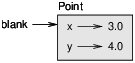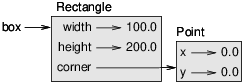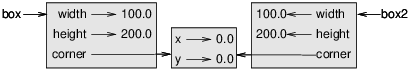# # 第十五章 类和对象

## # 15.1 用户自定义类型

• 我们可以把坐标存储成两个单独的值，x 和 y。

• 还可以把坐标存储成列表或者元组的元素。

• 还可以创建一个新的类型来用对象表示点。

``````    class Point:
"""Represents a point in 2-D space."""
``````
1
2

``````
>>> Point
<class '__main__.Point'>
``````
1
2
3

``````    >>> blank = Point()
>>> blank
<__main__.Point object at 0xb7e9d3ac>
``````
1
2
3

## # 15.2 属性

``````    >>> blank.x = 3.0
>>> blank.y = 4.0
``````
1
2

『Attribute』作为名词的发音要把重音放在第一个音节，而做动词的时候是重音放到第二音节。Figure 15.1: Object diagram.

``````
>>> blank.y
4.0
>>> x = blank.x
>>> x
3.0
``````
1
2
3
4
5
6

``````
>>> '(%g, %g)' % (blank.x, blank.y)
'(3.0, 4.0)'
>>> distance = math.sqrt(blank.x**2 + blank.y**2)
>>> distance
5.0
``````
1
2
3
4
5
6

``````    def print_point(p):
print('(%g, %g)' % (p.x, p.y))
``````
1
2

print_point 这个函数就接收了一个点作为参数，然后显示点的数值位置。你可以把刚刚那个 blank 作为参数传过去来试试：

``````    >>> print_point(blank)
(3.0, 4.0)
``````
1
2

## # 15.3 矩形

• 确定矩形的一个顶点（或者中心）所在位置，还有宽度和高度。

• 确定对角线上的两个顶点所在位置。

``````
class Rectangle:
"""Represents a rectangle.
attributes: width, height, corner.
"""
``````
1
2
3
4
5

``````    box = Rectangle()
box.width = 100.0
box.height = 200.0
box.corner = Point()
box.corner.x = 0.0
box.corner.y = 0.0
``````
1
2
3
4
5
6Figure 15.2: Object diagram.

## # 15.4 多个实例作返回值

``````    def find_center(rect):
p = Point()
p.x = rect.corner.x + rect.width/2
p.y = rect.corner.y + rect.height/2
return p
``````
1
2
3
4
5

``````    >>> center = find_center(box)
>>> print_point(center)
(50, 100)
``````
1
2
3

## # 15.5 对象可以修改

``````    box.width = box.width + 50
box.height = box.height + 100
``````
1
2

``````    def grow_rectangle(rect, dwidth, dheight):
rect.width += dwidth
rect.height += dheight
``````
1
2
3

``````    >>> box.width, box.height
(150.0, 300.0)
>>> grow_rectangle(box, 50, 100)
>>> box.width, box.height
(200.0, 400.0)
``````
1
2
3
4
5

## # 15.6 复制

``````
>>> p1 = Point()
>>> p1.x = 3.0
>>> p1.y = 4.0
>>> import copy
>>> p2 = copy.copy(p1)
``````
1
2
3
4
5
6

p1和 p2包含的数据是相同的，但并不是同一个点对象。

``````    >>> print_point(p1)
(3, 4)
>>> print_point(p2)
(3, 4)
>>> p1 is p2
False
>>> p1 == p2
False
``````
1
2
3
4
5
6
7
8

is 运算符表明 p1和 p2不是同一个对象，这就是我们所预料的。但你可能本想着是==运算符应该得到的是 True 因为这两个点包含的数据是一样的。这样的话你就会很失望地发现对于实例来说，==运算符的默认行为就跟 is 运算符是一样的；它也还是检查对象的身份，而不是对象的相等性。这是因为你用的是用户自定义的类型，Python 不值得如何去衡量是否相等。至少是现在还不能。

（译者注：==运算符的实现需要运算符重载，也就是多态的一种，来实现，也就是对用户自定义类型，需要用户自定义运算符，而不能简单地继续用内置运算符。因为自定义类型的运算是 Python 没法确定的，得用户自己来确定。）

``````    >>> box2 = copy.copy(box)
>>> box2 is box
False
>>> box2.corner is box.corner
True
``````
1
2
3
4
5Figure 15.3: Object diagram.

``````
>>> box3 = copy.deepcopy(box)
>>> box3 is box
False
>>> box3.corner is box.corner
False
``````
1
2
3
4
5
6

box3和 box 就是完全隔绝开，没有公用内嵌对象，彻底不会相互干扰的两个对象了。

## # 15.7 调试

``````
>>> p = Point()
>>> p.x = 3
>>> p.y = 4
>>> p.z
AttributeError: Point instance has no attribute 'z'
``````
1
2
3
4
5
6

``````    >>> type(p)
<class '__main__.Point'>
``````
1
2

``````
>>> isinstance(p, Point)
True
``````
1
2
3

``````    >>> hasattr(p, 'x')
True
>>> hasattr(p, 'z')
False
``````
1
2
3
4

hasattr 的第一个参数可以是任意一个对象；第二个参数是一个字符串，就是要判断是否存在的属性名字。

``````    try:
x = p.x
except AttributeError:
x = 0
``````
1
2
3
4

## # 15.8 Glossary 术语列表

class: A programmer-defined type. A class definition creates a new class object.

class object: An object that contains information about a programmer-defined type. The class object can be used to create instances of the type.

instance: An object that belongs to a class.

instantiate: To create a new object.

attribute: One of the named values associated with an object.

embedded object: An object that is stored as an attribute of another object.

shallow copy: To copy the contents of an object, including any references to embedded objects; implemented by the copy function in the copy module.

deep copy: To copy the contents of an object as well as any embedded objects, and any objects embedded in them, and so on; implemented by the deepcopy function in the copy module.

object diagram: A diagram that shows objects, their attributes, and the values of the attributes.